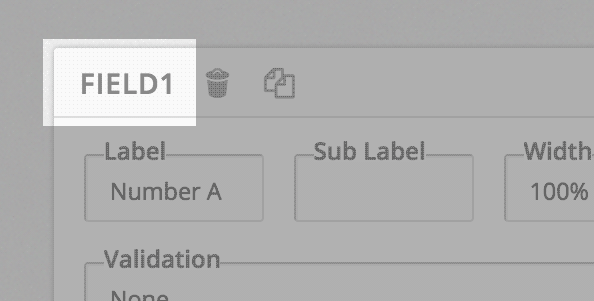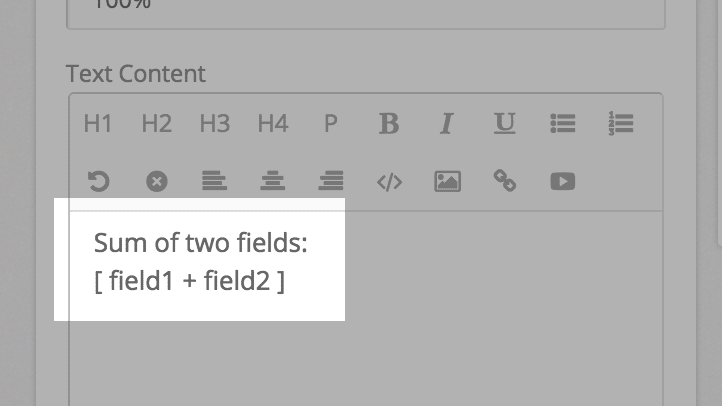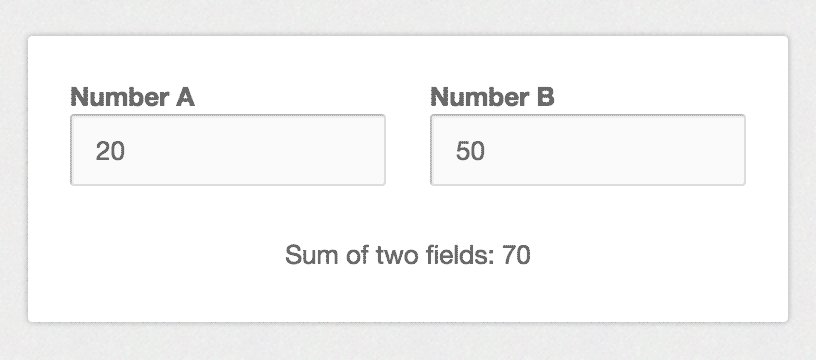# How To Use Math Formulas

## Simple Example

Add two One Line Input fields in your form. Note the Field IDs of both fields:Now add a Custom Text field to the form. In the Text Content area, type the formula: [ field1 + field2 ]You can click on Preview. Our form would look like this:You can type two numbers in the fields, and our text field will automatically calculate the value, and show it.

## Summary

You can use math formulas in the Text Content or Field Value columns of a Custom Text field.

A formula is enclosed in square brackets. Basic operators are allowed in math formulas: + – / * ( )

## Special Considerations

### Datepicker

You can use the math formula here to calculate the difference between two dates. If you have two date fields in your form, with fields IDs being field2 and field5, you can use this formula: [ field5 – field2 ]